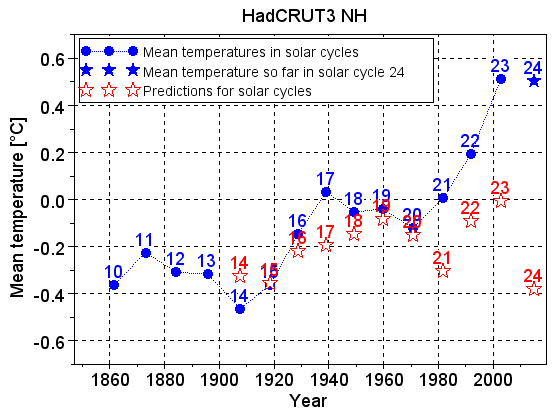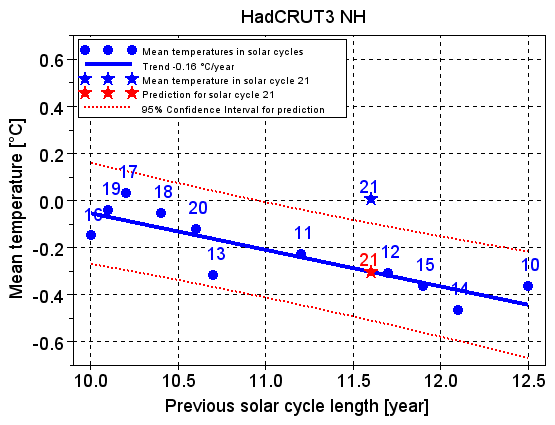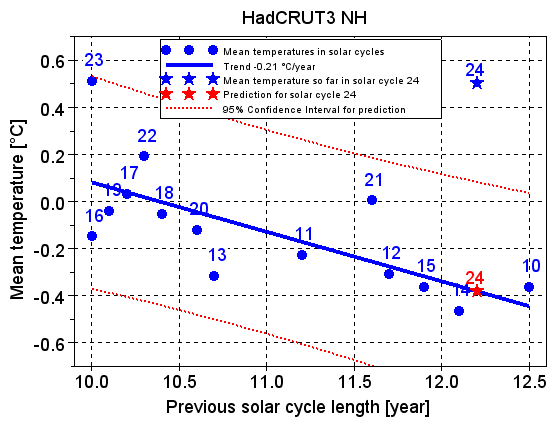# Solar Cycle Model fails to predict the recent warming

Jan-Erik Solheim, Kjell Stordahl and Ole Humlum have recently published two papers supporting the myth that the sun is behind the recent climate changes. They make a model based on variations in the lengths of the solar cycles. The model predicts that we are now in a decade with sharply falling temperatures.

However, as will be shown in this post, the model fails to keep up with the recent global warming. Temperatures fitted well with the model until the mid-1970s, but not later. It is therefore extremely unlikely that the prediction about sharply falling temperatures in this decade will be right.

The solar intensity varies by approximately 0.1% over a solar cycle. Both the variations and the average value of the intensity differ a little from one cycle to the next. These variations are a solar radiative forcing that affects the energy balance of the Earth. A solar cycle lasts on average for just over 11 years. The previous solar cycle, number 23, ended in November 2008 after having lasted for an unusually long time, well over 12 years. The current solar cycle 24 will probably last for the rest of the decade.

Jan-Erik Solheim, Kjell Stordahl and Ole Humlum (hereafter SSH) find a relationship between the mean temperature in a solar cycle and the length of the previous solar cycle [1, 2], which we will refer to as the Solar Cycle Model: A long solar cycle is followed by a solar cycle with a low temperature, and a short solar cycle is followed by a solar cycle with a high temperature.

When a solar cycle has ended, its length is known, and the model can predict the temperature in the next solar cycle. Due to the long solar cycle 23, the model predicts that we are now in a solar cycle with sharply falling temperatures.

In this blog post, observed mean temperature is the average of the observed global surface temperatures in a solar cycle, and predicted temperature is the prediction by the Solar Cycle Model for the mean temperature in a solar cycle. A prediction is calculated based on the observed mean temperatures in the previous solar cycles and the lengths of the previous solar cycles.

### Results based on temperatures in the northern hemisphere

SSH concentrate on some local temperature series from the northern hemisphere. The HadCRUT3 NH temperature series contains the combined land and sea surface temperatures for the entire northern hemisphere. We first examine how this series matches the Solar Cycle Model.

Figure 1 shows the observed mean temperatures in solar cycles 10 to 23 as blue circles, and the mean temperature observed so far in the current solar cycle 24 as a blue star. It also shows the predicted temperatures in solar cycles 14 to 24 as red stars. The horizontal positions of the temperatures are in the middle of the solar cycles that they represent.Figure 1. The observed and predicted temperatures up to now.

Figure 1 shows that the observed mean temperatures in solar cycles 21 to 23 are much higher than predicted.

SSH predict sharply falling temperatures in the current solar cycle 24. But the mean temperature observed so far in solar cycle 24 is much higher than predicted. Solar cycle 24 still has many years to go, and the situation may of course change. But given the failures of the predictions for cycles 21 to 23, and the high mean temperature observed so far in cycle 24, the model's prediction for cycle 24 is extremely unlikely to be right.

Figure 2 shows the temperatures in solar cycles 10 to 21 as a function of the length of the previous solar cycle. Solar cycle 21 ended in October 1986.Figure 2. The observed mean temperatures up to and including solar cycle 21, and the prediction for cycle 21, as a function of the length of the previous solar cycle.

The blue trend line sloping downwards in Figure 2 is the best fit to the blue circles, which show the observed mean temperatures in solar cycles 10 to 20. The trend is calculated with linear regression analysis. The observed mean temperatures fit well with the Solar Cycle Model when they are close to the trend line, and they do not fit well when they are far away from it.

Solar cycle 20 lasted for 11.6 years, and the prediction for solar cycle 21 is therefore on the trend line at the horizontal value 11.6 years. The dotted red lines show the 95% confidence interval (uncertainty range) for the prediction.

The observed mean temperature in solar cycle 21 is displayed as a blue star in Figure 2. It is far above the upper limit of the 95% confidence interval around its prediction. According to the model, there is only a 0.41% probability of measuring such a high mean temperature in solar cycle 21.

Solar cycle 20 ended in June 1976. Figure 2 shows that the temperatures measured before the mid-1970s fit very well with the Solar Cycle Model, but that temperatures in the first solar cycle after the mid-1970s do not. Figure 2 shows when the Solar Cycle Model collapsed with respect to the ability to predict future temperatures.

The current solar cycle 24 started in December 2008, and it will probably last for the rest of the decade. Figure 3 shows how the Solar Cycle Model predicts its temperature, just as Figure 2 did for cycle 21.Figure 3. The observed mean temperatures up to now, and the prediction for cycle 24, as a function of the length of the previous solar cycle.

Due to the high temperatures in solar cycles 21 to 23, the blue circles in Figure 3 are no longer close to the blue trend line. The observed mean temperatures in solar cycles 10 to 23 as a whole do not fit with the Solar Cycle Model, causing the uncertainty of the model to increase and thereby the confidence intervall around the prediction to expand. Despite the greatly expanded confidence interval, the mean temperature observed so far in solar cycle 24 is far above the upper limit of the 95% confidence interval around its prediction.

### Results based on other temperature series

This blog post concentrates on the temperatures in the northern hemisphere, and it therefore shows results based on the HadCRUT3 NH temperature series. Results based on the global HadCRUT3, NASA GISS and NCDC temperature series are about the same as the results based on HadCRUT3 NH; i.e. the observed mean temperatures match the predictions until the mid-1970s, but not later. SSH also use the HadCRUT3 NH temperature series, but they place more emphasis on some local temperature series.

This blog post is a basic version of a longer article. It shows that the median of the results based on the local temperature series also diverges from the Solar Cycle Model predictions after the 1970s, in about the same way as does the results based on the HadCRUT3 NH temperature series. The longer article also contains more explanations, plots and statistics.

### Conclusion

If there is a real, physical reason why temperatures fitted so well with the Solar Cycle Model until the mid-1970s, that reason must be a solar radiative forcing. If so, this forcing must still be present after the mid-1970s, although it no longer dominates. Another forcing, or several, must have become dominant. My analysis says nothing about what the new dominant forcing(s) may be. But it is natural to think of the human-induced forcings, which many scientists claim became dominant in the 1970s.

Skeie et al  show that the sum of the various human-induced forcings first became positive in the 1970s. Before 1970, the sum was small and the sign varied. After 1970, the sum has increased steadily up to a substantial positive value in 2010. This is the probable explanation why there is an increasing gap between the Solar Cycle Model's predictions and the observed mean temperatures after the middle of the 1970s.

### Acknowledgements

A special thanks to Christian Moe for valuable comments and for language editing.

### References

1. Solar Activity and Svalbard Temperatures
Jan-Erik Solheim, Kjell Stordahl and Ole Humlum.

2. The long sunspot cycle 23 predicts a significant temperature decrease in cycle 24
Jan-Erik Solheim, Kjell Stordahl and Ole Humlum.

Posted by Hans Petter Jacobsen on Wednesday, 19 December, 2012The Skeptical Science website by Skeptical Science is licensed under a Creative Commons Attribution 3.0 Unported License.